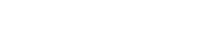# ISA Certified Automation Professional (CAP) program

• CAP

## CAP question

The greatest probability of error being introduced into the “open-loop” method of tuning comes from determining the:

A. process time constant
B. reaction equilibrium
D. system gain

The answer is C, actual dead time. In open-loop methods of tuning, like Ziegler-Nichols, the dead time derived from the open-loop response is a part of the calculation of all of the tuning parameters for P-only, PI, and PID (proportional, integral, derivative) controllers. Often, the dead time is small (flow and pressure loops), and defining the quantity of the dead time from response curves can be tricky. Depending on how the tangent to the response curve is drawn, a very small dead time may be off by a factor of two or more, leading to an inaccurate calculation of the tuning parameters. Because the dead time is in the denominator of the equation for the proportional gain, the difference between 0.1 and 0.2 minutes can be insignificant on the response plot but would yield gains that differ by a factor of two.

Because the values of the process time constant and system gain are usually quite significant, those quantities can be determined with open-loop methods fairly accurately.

Reference: Sands, Nicholas P. & Verhappen, Ian, A Guide to the Automation Body of Knowledge, Third Edition, ISA Press, 2019.